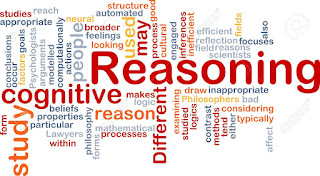# Reasoning Quiz - IBPS PO, Clerk, RRBMentor for Bank Exams
Welcome to Mentor for Bank Exams. Here I am presenting the Reasoning Quiz of clerical level. This will be helpful in the preparation of upcoming bank exams like IBPS PO Prelims, Clerk Prelims and RRB Prelims and Mains. Kindly Make use of these!!!
Directions (1 – 5): Study the following arrangement carefully and answer the questions given below:
4 X A \$ 3 1 Y Z 8 @ W T 6 % 7 U P & Q 0 5 R # M N O € K 2
1. If all the vowels from the above arrangement are dropped, which of the following will be the 10th from the left end?
a) @
b) T
c) Q
d) W
e) %
2. Four of the following five are alike in a certain way based on their positions in the above arrangement and so form a group. Which is the one that does not belong to that group?
a) \$3A
b) NOM
c) P%U
d) @W4
e) 5E#
3. How many such alphabets are there in the above arrangement, each of which is immediately preceded by a symbol and immediately followed by a number?
a) One
b) Two
c) Three
d) Four
e) None
4. How many such numbers are there in the above arrangement, each of which are immediately preceded by a symbol and immediately followed by a vowel?
a) Five
b) Four
c) Three
d) Two
e) One
5. In which of the following combinations the first element lies equidistant from the second and the third element in the above arrangement?
a) 1 \$ Y
b) 4 Z W
c) # M O
d) A 4 3
e) 5 R M
Directions (6 – 10): Study the following arrangement carefully and answer the questions below:
Z 2 X % D A # G * H 3 E J \$ 4 B 1 @ S T I 5 & U K W 8 P Q 7 Y 6
6. How many such consonants are there in the above arrangement, each of which is immediately preceded by a number but not immediately followed by a consonant?
a) None
b) One
c) Two
d) Three
e) More Than Three
7. How many such symbols are there in the above arrangement, each of which is immediately preceded by a consonant and immediately followed by a number?
a) One
b) Zero
c) Two
d) Three
e) More than three
8. How many such vowels are there in the above arrangement, each of which is immediately preceded by a symbol and immediately followed by a consonant?
a) None
b) One
c) Two
d) Three
e) More than three
9. Which of the following is the eighth to the right of the sixteenth from the right end of the above arrangement?
a) 5
b) H
c) K
d) 1
e) More than three
10. Four of the following are alike in a certain way based on their positions in the above arrangement and so form a group. Which is the one that does not belong to that group?
a) 9GD
b) J43
c) WPU
d) DX9
e) SI1